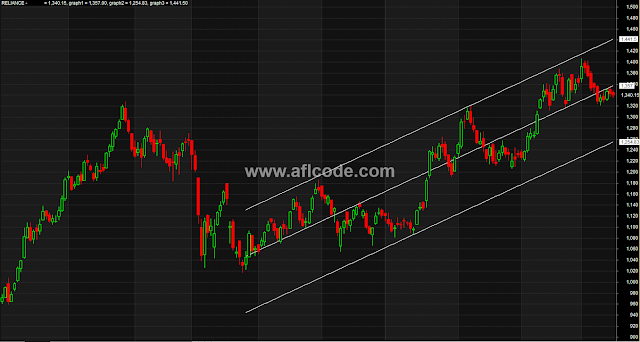### Linear Regression Lines And BandsLinear Regression Lines And Bands

```//www.aflcode.com

N = LastValue(LLVBars(Low,190));   // lookback period  - can be set by the user if necessary
Start = 1;

X = Cum(Start);    // Set up the x cordinate array of the Linear Regression Line
Y = Close;         // Set the y co-ordinate of the Linear Regression line

/* Calculate the slope (bconst) and the y intercept (aconst) of the line */

SUMX    = LastValue(Sum(X,N));
SUMY    = LastValue(Sum(Y,N));
SUMXY   = LastValue(Sum(X*Y,N));
SUMXSqd = LastValue(Sum(X*X,N));
SUMSqdX = LastValue(SUMX * SUMX);

bconst  = (N * SUMXY - SUMX * SUMY)/(N * SUMXSqd - SUMSqdX);
aconst  = (SUMY - bconst * (SUMX))/N;

/* Force the x value to be very negative so the graph does not apear before the lookback period */

Domain =  IIf ( X > LastValue(X) - N, 1 , -1e10);
Xvar = X * Domain;

/* Linear Regression Line */

Yvar = aconst + bconst * Xvar;

/* Plot the graphs */

GraphXSpace = 10;
MaxGraph=4;

/* Candle chart */
Graph0 = Close;
Graph0Style = 64;
Graph0Color = 1;

/* Linear Regression Lines */
Graph1 = Yvar;
Graph2 = Yvar - LastValue(HHV(Yvar - Low,N));
Graph3 = Yvar + LastValue(HHV(High - Yvar,N));

Graph1Style = 1;
Graph1Color = 2;
Graph2Style = 1;
Graph2Color = 2;
Graph3Style = 1;
Graph3Color = 2;
```

Previous
Next Post »